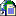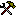Mathematics
Stuff about algorithms; explanations, implementations, and pitfalls.Explaining the Levenberg-Marquart methods for solving equations This article explains the mathematical foundation for Levenberg-Marquart methods, but has no source code example (not even in Fortran). Good for understanding.FreeVBCode This site is a large and well sorted collection of source code. The code pieces range from banal to excellent, and should satisfy all levels of expertise.MatrixVBUsing Newtonian Approximation to solve equations If you want to solve polynominal equations, you can use the Newton-Raphson method of approximating the solutions. This routine takes parameters too.